## Types of Single Phase Synchronous Motor:

There are two types of single phase synchronous motor, namely,

• Reluctance Motor
• Hysteresis Motor

### Construction of Reluctance Motor and Torque Speed Characteristics:

The reluctance motor, in general, results wherever the stator produces a rotating field in space and the rotor is noncylindrical such that the reluctance of the magnetic path offered by the rotor to the rotating field is a function of the space angle. The origin of the reluctance torque lies in the tendency of the rotor to align itself in the minimum reluctance position with respect to the synchronously rotating flux of the forward field. The motor is made self-starting by the induction principle by providing short-circuited copper bars in the projecting parts of the rotor.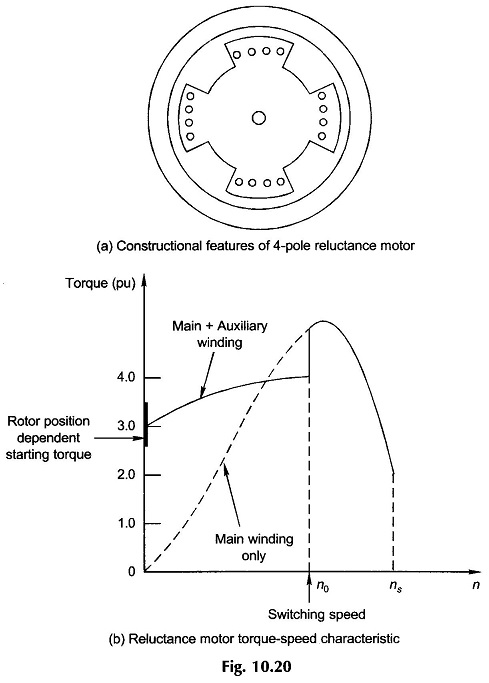In a single-phase reluctance motor the rotating field can be produced by any of the phase-splitting methods discussed above. The salient-pole structure is given to the rotor by removing some of the teeth of an induction motor rotor as shown in Fig. 10.20(a). The remaining teeth carry short-circuited copper bars to provide the starting induction torque. After starting the rotor reaches near synchronous speed by induction action and is pulled into synchronism during the positive half-cycle of the sinusoidally varying synchronous torque. This would only be possible if the rotor has low inertia and the load conditions are light. The torque-speed characteristic of a typical reluctance motor with induction start is given in Fig. 10.20(b). As seen from this figure the starting torque is highly dependent upon the rotor position because of the projecting nature of the rotor. This phenomenon is known as cogging. For satisfactory synchronous motor performance, the frame size to be used must be much larger than that for a normal single phase induction motors. This accounts for the high value of starting torque indicated in Fig. 10.20(b).

### Hysteresis Motor:

Another types of Single Phase Synchronous Motor is the hysteresis motor. The stator of a hysteresis motor is wound with main and auxiliary windings with a permanently connected capacitor for phase splitting. The capacitor is selected to create balanced 2-phase conditions. The rotor is a smooth solid cylinder of hard steel (this has high hysteresis loss) and does not carry any winding (no rotor bars).

Both the stator windings are distributed such as to create a rotating field with as nearly a sinusoidally space distribution as possible; this is necessary to keep down iron-loss due to space harmonics of the field.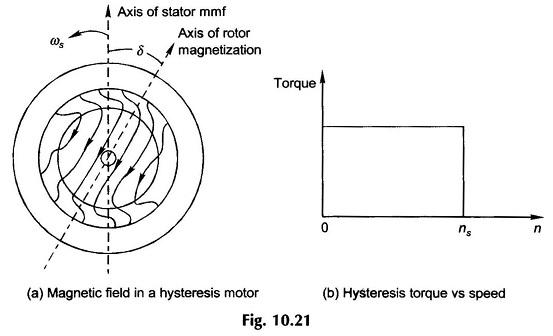The phenomenon of hysteresis causes the rotor magnetization to lag behind the stator-created mmf wave. As a consequence, the rotor flux lags by angle δ the stator mmf axis. Figure 10.21(a) shows the magnetic condition in the motor at any instant. As the angle δ is hysteresis-dependent, it remains constant, at all rotor speeds. The interaction torque (hysteresis torque) between stator and rotor fields therefore is constant at all speeds (Fig. 10.21(b)). Under the influence of the hysteresis torque the rotor accelerates smoothly and finally runs at synchronous speed with angle δ getting adjusted to the load torque. This is a contrast to the “pull-in” phenomenon in a reluctance motor when it synchronizes. Constancy of the hysteresis torque is demonstrated by the derivation below.

The hysteresis loss is expressed as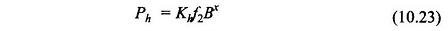where

• B = maximum flux density,
• f2 = sf = rotor frequency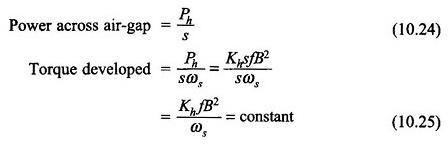Another component of torque caused by eddy-current loss is simultaneously created in the motor. This can be derived as: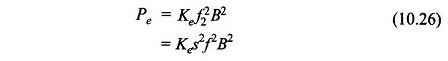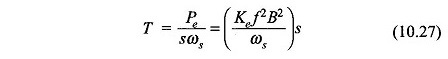As per Eq. (10.27), the eddy-current torque is highest at start and reduces linearly with slip vanishing at synchronous speed. This torque component aids the hysteresis torque at starting, endowing excellent starting characteristic to the hysteresis motor.

The hysteresis motor has a low noise figure compared to the Single phase induction motor such that the load runs at uniform speed. This is because it operates at one speed (synchronous) and nearly balanced 2-phase conditions are not disturbed (as they would in the induction motor when the slip changes with load). Further, a smooth (unslotted) rotor greatly aids in low noise performance of this motor. Multispeed operation is easily possible by arranging pole changing of stator windings; the rotor being unwound reacts to create the same number of poles as the stator. As already pointed out, the motor has excellent starting characteristics (starting torque equal to running torque). Therefore, it is well-suited to accelerate high-inertia loads.

Scroll to Top